# Text Analysis & Deep Learning¶

In :
import numpy as np
import pandas as pd
import torch
from torch import nn
from functools import partial
from tqdm import tqdm

In :
# configure matplotlib output
import matplotlib.pyplot as plt
import matplotlib as mpl
mpl.style.use('config/clean.mplstyle') # this loads my personal plotting settings
col = mpl.rcParams['axes.prop_cycle'].by_key()['color']
%matplotlib inline

In :
# if you have an HD display
%config InlineBackend.figure_format = 'retina'

In :
# some warnings can get annoying
import warnings
warnings.filterwarnings('ignore')


## PyTorch (torch)¶

Now we're moving on to torch, a very popular machine learning framework, probably the most popular nowadays. Like jax, torch implements an interface very similar to that of numpy. However, instead of being basically a drop in replacement, there are some differences and all the functions are exposed directly in the top-level torch module.

First, arrays aren't called array anymore, they're called tensor. As with jax, you need to be a little more careful about array types, as most things are done in 32-bit, and arrays always have a device that they are located on, which can be either cpu or cuda most of the time.

In :
x = torch.tensor([1.0, 2.0, 3.0])
print(x.device, x.dtype)
x

cpu torch.float32

Out:
tensor([1., 2., 3.])

As an example, in numpy and jax, you can take the mean of an integer or boolean array. However, in torch this will throw an error, as mean would then return a number of a different type (float). Here's an example

In :
try:
b = torch.tensor([1, 2, 3])
b.mean()
except Exception as e:
print(e)

mean(): could not infer output dtype. Input dtype must be either a floating point or complex dtype. Got: Long


We'll want to find the mean of some boolean arrays below, so let's define a convenience function. This uses the to function, which allows you to mutate properties of a tensor such as device or dtype. Also note that below we use chaining of functions on tensors, which is quite common in torch world, rather than top-level torch functions.

In :
bool_mean = lambda x: x.to(dtype=torch.float).mean()


### Neural Classifier¶

First let's reimplement the neural classifier model from last lecture in torch. The data generation is nearly identical to before. Note that categ is a boolean but is encoded as an int here. After generating the raw data, we pass it to TensorDataset to create a single dataset object. This way we don't have to carry around multiple tensors. Then we pass this to DataLoader, which takes care of things like randomly shuffling the data and making batches. If you use the dataloader object as an iterator, it will return batches of space/categ pairs.

In :
# simple hyperplane model
dim = 100
true_beta0 = 0.0
true_beta1 = 1.0*torch.ones(dim)

In :
# generate raw data
N = 10000
space = torch.randn(N, dim)
rando = torch.tensor(np.random.logistic(size=N))
categ = (true_beta0 + space @ true_beta1 + rando > 0).to(dtype=torch.long)

In :
# make dataset and loader
dataset = torch.utils.data.TensorDataset(space, categ)


Now let's create the actual model, which is a Module object. This looks very similar to the flax notation. In this framework, any setup like layer creation is done in __init__, while the actual prediction is done in the forward function. Notice that here we also return a pair of logits that defines the probabilities of the respective outcomes (0 and 1). Sadly, we don't have trees in torch world, so we just make a tensor of stats.

In :
# same neural as a module
class Neural(nn.Module):
def __init__(self, hid=2):
super().__init__()
self.linear0 = nn.Linear(dim, hid)
self.linear1 = nn.Linear(hid, 2)

def forward(self, x):
x = self.linear0(x)
x = torch.sigmoid(x)
x = self.linear1(x)
return x

In :
def neural_stats(logits, y):
predict = logits[:, 1] >= logits[:, 0]
target = y == 1
bool_mean(predict == target),
bool_mean(predict & ~target),
bool_mean(~predict & target),
])


Now let's run it! There's a lot going on in this cell. You can see it's not so simple and elegant as in jax. Some of this is for the sake of performance. We don't have to do anything like jit here, but things are still pretty fast, and the cost is slightly clunkier notation. There are two primary differences below. One is this code block:

optim.zero_grad()
loss.backward()
optim.step()


When we run model and bce_loss, this is the "forward" pass where we compute values. To get the gradients, we have to a do a "backward". That's what's happening here. We zero out the stored gradients, accumulate them with a backward pass, then take a step based on those gradients. We run the training loop for 25 "epochs", which means we run it over the entire dataset 25 times.

The other difference is the logits.detach() command. This actually isn't truly necessary, we could have just written logit. All that detach does it tell it to stop tracking gradient information for that calculation, as we don't need the gradients for the neural_stats values. Similarly, the numpy command converts a tensor into the analgous numpy array.

In :
# make model and optimizer
model = Neural()
optim = torch.optim.RMSprop(model.parameters(), lr=0.01)

# define loss function
bce_loss = nn.CrossEntropyLoss()

# track history
history = []

# go for many iterations
for i in range(25):
# accumulate stats per batch
nbatch = 0
stats = torch.zeros(3)

# iterate over batches
# compute loss
logits = model(x)
loss = bce_loss(logits, y)

# update params
loss.backward()
optim.step()

nbatch += 1
stats += neural_stats(logits.detach(), y)

# make it a mean
stats /= nbatch
history.append(stats)

# turn history into dataframe
history = pd.DataFrame(
torch.stack(history).numpy(),
columns=['accuracy', 'false_pos', 'false_neg']
)
history.plot(subplots=True, layout=(1, 3), figsize=(12, 3));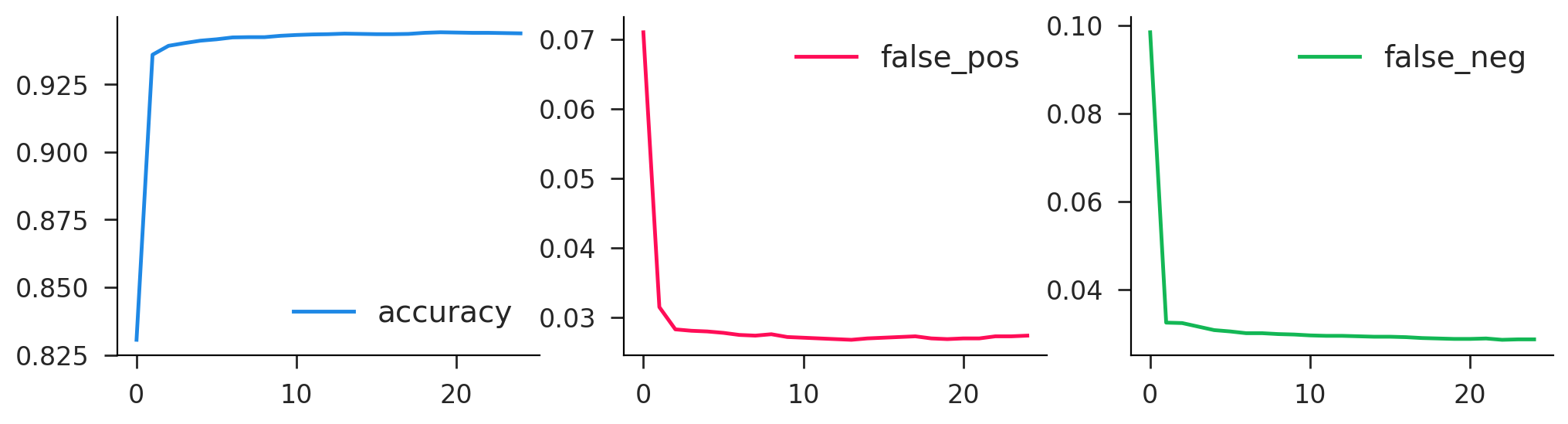Okay, time to make some money!! We're going to generate some data on "returns", which are just random normals, which may or may not have a predictable component. There is a single security, so our choice is what fraction of our money to invest in each period. We're assuming we can only take "long" positions, so this is a number $s \in [0,1]$. Our input data is the history of the past $L$ realizations of returns. From a single series of length $T$, we can turn this into a set of $T-L$ overlapping history/outcome pairs.

In :
# parameters
N = 1000 # number of training series
M = 100 # number of validation series
T = 20 # total time length
L = 4 # history window length
K = T - L # number of subseries

In :
# generate overlapping sequences with l-length history and single outcome
def generate_sequences(vec, l):
n, t = vec.shape
k = t - l
index0 = torch.arange(l).unsqueeze(0) + torch.arange(k).unsqueeze(1)
index = index0.flatten().expand(n, k*l)
series = torch.gather(vec, 1, index).reshape(n*k, l)
target = vec[:, l:].reshape(n*k)
return series, target


The model is a two layer neural network as before. The output for each observation is a scalar in $[0,1]$, which we accomplish with the sigmoid on output. Note that that final squeeze operation makes sure it's a scalar rather than a length-1 tensor.

In :
# simple neural trader (long only)
def __init__(self, lag=4, hid=5):
super().__init__()
self.linear0 = nn.Linear(lag, hid)
self.linear1 = nn.Linear(hid, 1)

def forward(self, x):
x = self.linear0(x)
x = torch.sigmoid(x)
x = self.linear1(x)
x = torch.sigmoid(x)
return x.squeeze()


Now it is time to train. This is all quite similar to before. Notice the objective here is just the product of the position recommendation and the realized returns (averaged over the batch). One addition you'll notice is we wrap the epoch loop in the mysterious tqdm. This will function the same in the code but will also print out a neat little progress bar. Also, there are new model.train() and model.eval() commands. These are unecessary in the basic case, but we'll need them later.

In :
def neural_trader(model, train_data, valid_data, lr=0.01, epochs=25, batch_size=1000):
valid_x, valid_y = valid_data.tensors

# make model and optimizer
optim = torch.optim.RMSprop(model.parameters(), lr=lr)

# track history
history = []

# go for many iterations
for i in tqdm(range(epochs)):
n_batch = 0
train_stats = torch.zeros(2)

# iterate over batches
model.train()
# compute loss
recom = model(batch_x)
retvrn = recom*batch_y
loss = -retvrn.mean()

# update params
loss.backward()
optim.step()

# training stats
n_batch += 1
train_stats += torch.tensor([recom.detach().mean(), retvrn.detach().mean()])

# average training
train_stats /= n_batch

# validation stats
model.eval()
valid_recom = model(valid_x)
valid_retvrn = valid_recom*valid_y
valid_stats = torch.tensor([valid_recom.detach().mean(), valid_retvrn.detach().mean()])

# make it a mean
stats = torch.cat([train_stats, valid_stats])
history.append(stats)

# turn history into dataframe
names = ['train_recom', 'train_return', 'valid_recom', 'valid_return']
return pd.DataFrame(torch.stack(history).numpy(), columns=names)


### Overfitting¶

Now let's train this on some random data. Any returns we get should be purely noise, as these are just uncorrelated returns over time. You can see that we typically get 1-2% returns on the training data, while those in the validation set are over lower, but in general are quite noisy. The other thing to note is that for many runs, after some point in the training process we see the validation return start to go down, while the training return continues to rise. This is a classic signature of overfitting.

In :
# generate training data
train_random_return = torch.randn(N, T)
train_random_series, train_random_target = generate_sequences(train_random_return, L)
train_random_data = torch.utils.data.TensorDataset(train_random_series, train_random_target)

# generate validation data
valid_random_return = torch.randn(M, T)
valid_random_series, valid_random_target = generate_sequences(valid_random_return, L)
valid_random_data = torch.utils.data.TensorDataset(valid_random_series, valid_random_target)

In :
model_base = NeuralTrader(lag=L, hid=5)
history = neural_trader(model_base, train_random_data, valid_random_data, epochs=500)
history.plot(subplots=True, layout=(2, 2), figsize=(10, 6));

100%|█████████████████████████████████████████████████████████████████| 500/500 [00:20<00:00, 24.24it/s]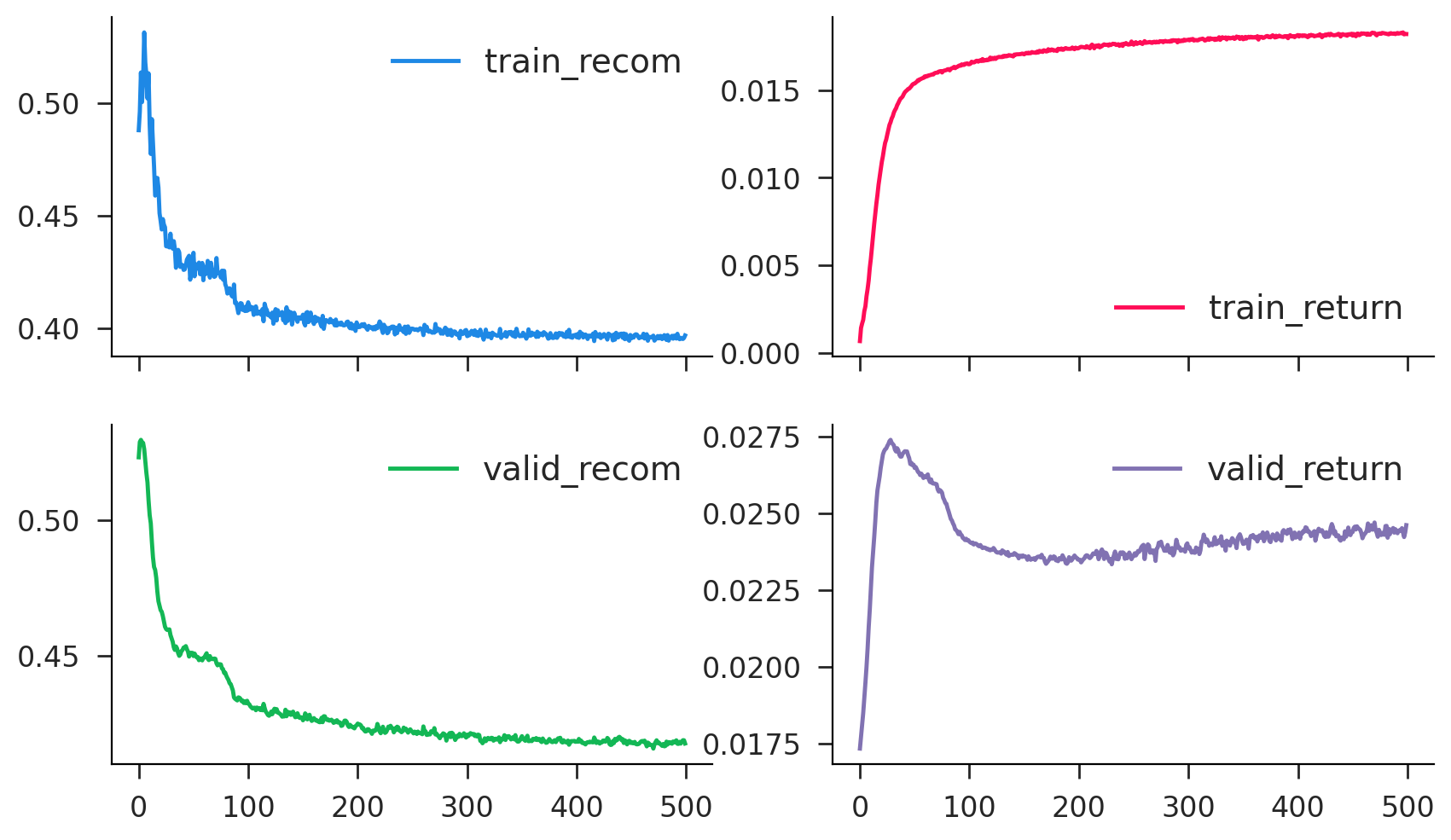### Real Signal (Momentum)¶

Now let's at least give ourselves a chance and add in some momentum (EMH violation alert!). Obivously this can happen in certain markets, and I think there's even an explanation in efficient markets relating to stochastic discount factors. Like it's okay as long as returns are uncorrelated with the SDF. Anyway, that doesn't matter here, we can simulate whatever the hell we want.

In :
# draw an MA(1) process with persistence ρ
def gen_ma1(N, T, ρ=0.5):
tvec = torch.arange(T).flip(0)
ρvec = (ρ**tvec).unsqueeze(0)
shocks = torch.randn(N, T)
values = (ρvec*shocks).cumsum(1)/ρvec
return values

In :
ρ = 0.2
train_moment_return = gen_ma1(N, T, ρ=ρ)
train_moment_series, train_moment_target = generate_sequences(train_moment_return, L)
train_moment_data = torch.utils.data.TensorDataset(train_moment_series, train_moment_target)
valid_moment_return = gen_ma1(M, T, ρ=ρ)
valid_moment_series, valid_moment_target = generate_sequences(valid_moment_return, L)
valid_moment_data = torch.utils.data.TensorDataset(valid_moment_series, valid_moment_target)

In :
model_moment = NeuralTrader(lag=L, hid=5)
history = neural_trader(model_moment, train_moment_data, valid_moment_data, epochs=250)
history.plot(subplots=True, layout=(2, 2), figsize=(10, 6));

100%|█████████████████████████████████████████████████████████████████| 250/250 [00:10<00:00, 24.50it/s]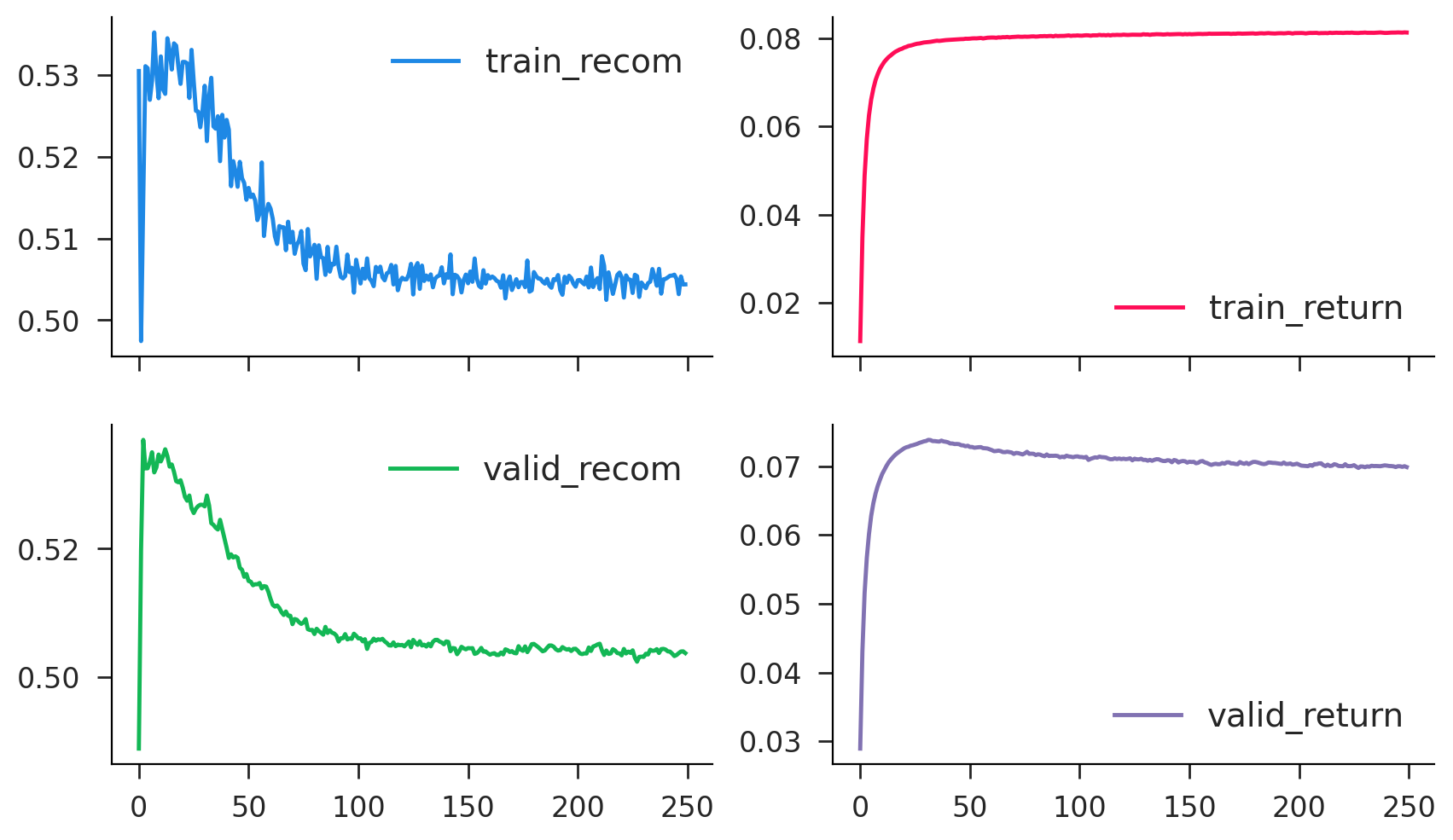### Dropout¶

We may be worried about what appears to be overfitting. The algorithm is just fitting noise. One popular approach to this is to use a method called "dropout" in which network connections are randomly severed during training (weights zeroed). This forces the algorithm to "diversify" and ideally reduces overfitting.

In :
# simple neural trader (long only)
def __init__(self, lag=4, hid=5, drop=0.5):
super().__init__()
self.linear0 = nn.Linear(lag, hid)
self.linear1 = nn.Linear(hid, 1)
self.dropout = nn.Dropout(drop)
self.drop = drop

def forward(self, x):
x = self.linear0(x)
x = torch.sigmoid(x)
x = self.dropout(x)
x = self.linear1(x)
x = torch.sigmoid(x)
return x.squeeze()

In :
model_random_dropout = DropoutTrader(lag=L, hid=5, drop=0.5)
history = neural_trader(model_random_dropout, train_random_data, valid_random_data, epochs=250)
history.plot(subplots=True, layout=(2, 2), figsize=(10, 6));

100%|█████████████████████████████████████████████████████████████████| 250/250 [00:10<00:00, 23.89it/s]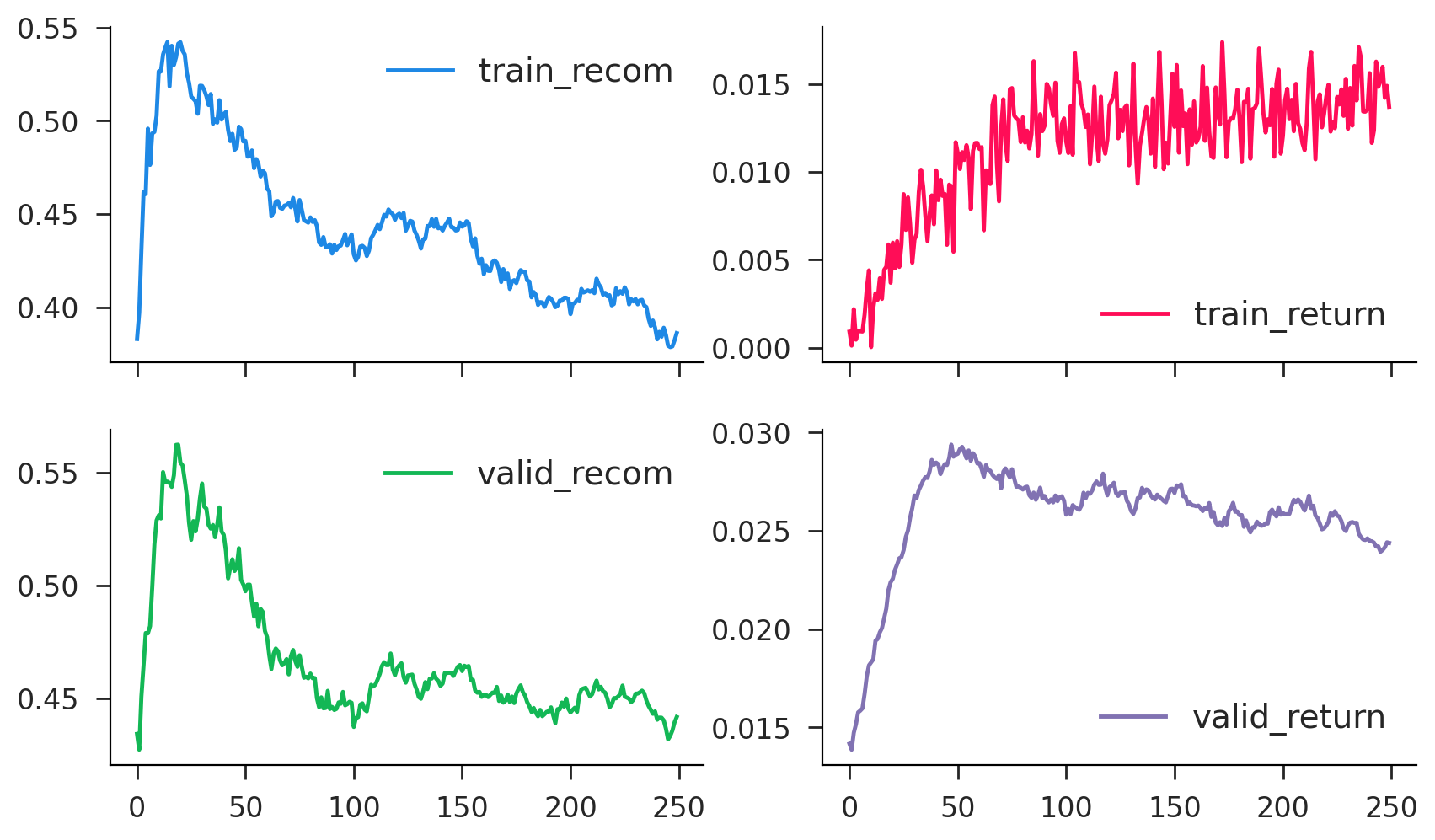In :
model_moment_dropout = DropoutTrader(lag=L, hid=5, drop=0.5)
history = neural_trader(model_moment_dropout, train_moment_data, valid_moment_data, epochs=250)
history.plot(subplots=True, layout=(2, 2), figsize=(10, 6));

100%|█████████████████████████████████████████████████████████████████| 250/250 [00:10<00:00, 22.87it/s]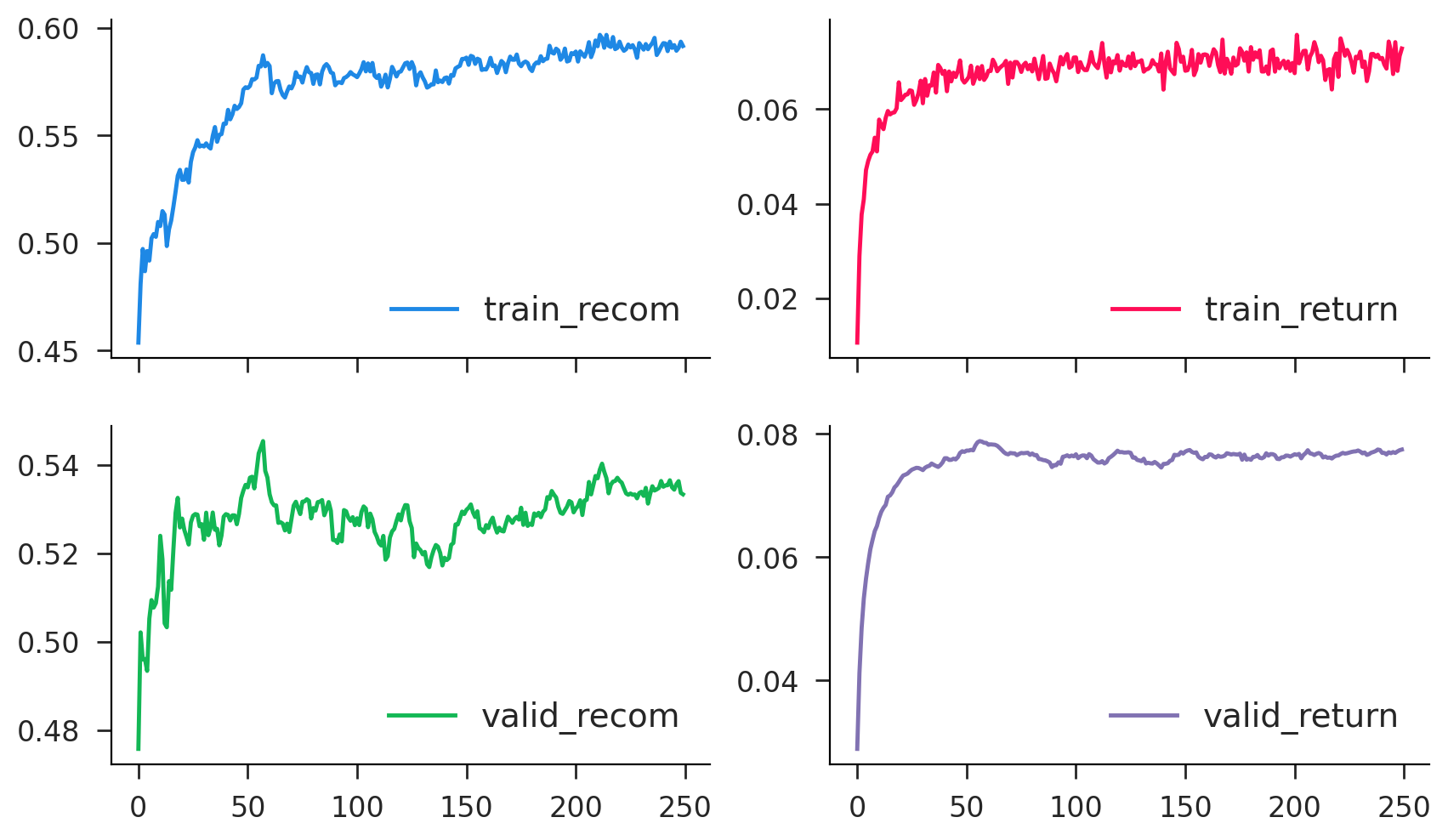## Text Analysis¶

In :
import re
import nltk
from sklearn.feature_extraction.text import CountVectorizer, TfidfVectorizer
from sklearn.manifold import TSNE

In :
def process_text(s):
# merge punctuation
s = re.sub(r'(;|\-\-)', r',', s)
s = re.sub(r'([[\-])', ' ', s)
s = re.sub(r'([,\.!\?"])', r' \1 ', s)
s = re.sub(r'\r', ' ', s)

# merge whitespace
s = re.sub(r'(?<!\n)\n(?!\n)', ' ', s)
s = re.sub(r'\n{2,}', '\n', s)
s = re.sub(r' {2,}', ' ', s)

# strip lines
s = re.sub(r'(^ +| +\$)', r'', s, flags=re.MULTILINE)
s = re.sub(r'\n', r' \\n ', s)

return s.lower()

In :
# download gutenberg corpus

[nltk_data] Downloading package gutenberg to /home/doug/nltk_data...
[nltk_data]   Package gutenberg is already up-to-date!

Out:
True
In :
# list available books
books = nltk.corpus.gutenberg.fileids()
names = [s.split('.') for s in books]
print(', '.join(names))

austen-emma, austen-persuasion, austen-sense, bible-kjv, blake-poems, bryant-stories, burgess-busterbrown, carroll-alice, chesterton-ball, chesterton-brown, chesterton-thursday, edgeworth-parents, melville-moby_dick, milton-paradise, shakespeare-caesar, shakespeare-hamlet, shakespeare-macbeth, whitman-leaves

In :
# get text of all books (corpus)
text = [nltk.corpus.gutenberg.open(f).read() for f in books]
print(text[117:443])

The pale Usher--threadbare in coat, heart, body, and brain; I see him
now.  He was ever dusting his old lexicons and grammars, with a queer
handkerchief, mockingly embellished with all the gay flags of all the
known nations of the world.  He loved to dust his old grammars; it
somehow mildly reminded him of his mortality.

In :
# get basic info about each text
length = np.array([len(x) for x in text])
info = pd.DataFrame({'name': names, 'length': length})
info

Out:
name length
0 austen-emma 887071
1 austen-persuasion 466292
2 austen-sense 673022
3 bible-kjv 4332554
4 blake-poems 38153
5 bryant-stories 249439
6 burgess-busterbrown 84663
7 carroll-alice 144395
8 chesterton-ball 457450
9 chesterton-brown 406629
10 chesterton-thursday 320525
11 edgeworth-parents 935158
12 melville-moby_dick 1242990
14 shakespeare-caesar 112310
15 shakespeare-hamlet 162881
16 shakespeare-macbeth 100351
17 whitman-leaves 711215
In :
text1 = [process_text(s) for s in text]
print(text1[:1000])

moby dick by herman melville 1851 etymology . ( supplied by a late consumptive usher to a grammar school ) the pale usher , threadbare in coat , heart , body , and brain , i see him now . he was ever dusting his old lexicons and grammars , with a queer handkerchief , mockingly embellished with all the gay flags of all the known nations of the world . he loved to dust his old grammars , it somehow mildly reminded him of his mortality . " while you take in hand to school others , and to teach them by what name a whale fish is to be called in our tongue leaving out , through ignorance , the letter h , which almost alone maketh the signification of the word , you deliver that which is not true . " , hackluyt " whale . . . . sw . and dan . hval . this animal is named from roundness or rolling , for in dan . hvalt is arched or vaulted . " , webster's dictionary " whale . . . . it is more immediately from the dut . and ger . wallen , a . s . walw ian , to roll , to wallow . " , richardson's d

In :
cv = CountVectorizer(stop_words='english')
counts = cv.fit_transform(text1)
nbook, nwrd = counts.shape
counts

Out:
<18x41757 sparse matrix of type '<class 'numpy.int64'>'
with 117238 stored elements in Compressed Sparse Row format>
In :
tfidf = TfidfVectorizer(stop_words='english')
vecs = tfidf.fit_transform(text1)
vecs

Out:
<18x41757 sparse matrix of type '<class 'numpy.float64'>'
with 117238 stored elements in Compressed Sparse Row format>
In :
counts.nnz/np.prod(counts.shape)

Out:
0.15597917049170731

### Text Similarity¶

In :
# check that we're really ℓ2 normalized
vecs.power(2).sum(axis=1).getA1()

Out:
array([1., 1., 1., 1., 1., 1., 1., 1., 1., 1., 1., 1., 1., 1., 1., 1., 1.,
1.])
In :
# get the full similarity matrix
sim = (vecs @ vecs.T).todense().getA() # not always feasible for big corpora
plt.imshow(np.sqrt(sim)); # sqrt enhances differences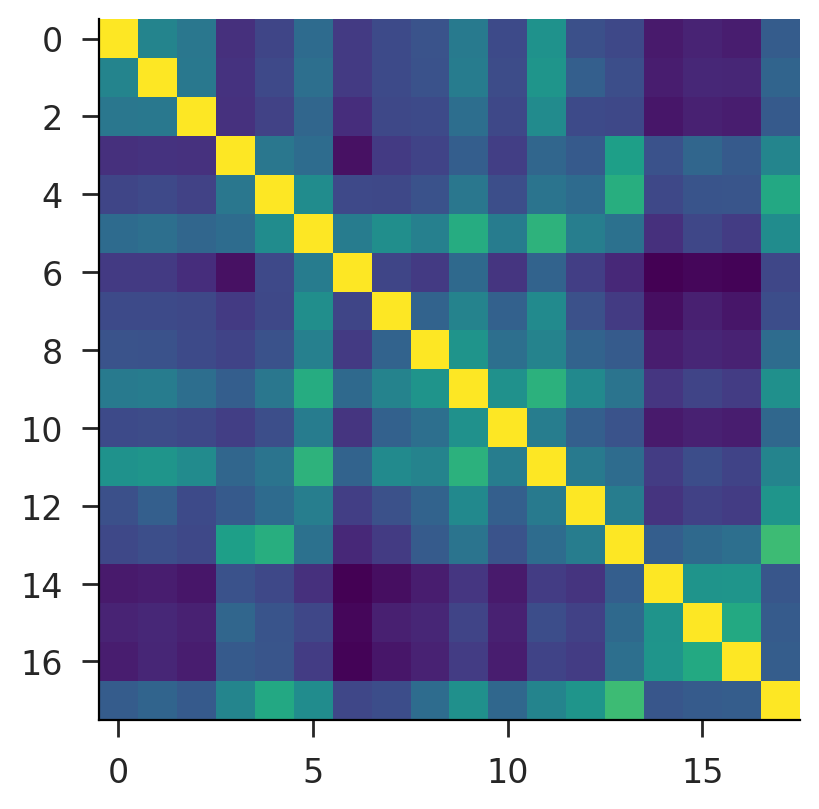In :
# get the most commonly used words
all_count = counts.sum(axis=0).getA1()
all_rank = np.argsort(-all_count)
all_words = cv.get_feature_names_out()
print('\n'.join([
f'{all_words[i]} — {all_count[i]}' for i in all_rank[:20]
]))

shall — 11682
said — 9429
unto — 9010
lord — 8590
thou — 6759
man — 5615
thy — 5609
god — 5287
thee — 4807
ye — 4674
come — 3642
like — 3468
came — 3337
day — 3326
king — 3149
did — 3121
little — 3065
know — 3057
house — 2900
good — 2899

In :
word_cdf = np.cumsum(all_count[all_rank])/np.sum(all_count)
word_gini = 2*(np.sum(word_cdf)/nwrd-0.5)
word_index = np.linspace(0, 1, nwrd)
pd.Series(word_cdf, index=word_index).plot(xlim=(0, 1), ylim=(0, 1))
plt.plot(word_index, word_index, linestyle='--', linewidth=1, c='k')
print(word_gini)

0.8576843343208485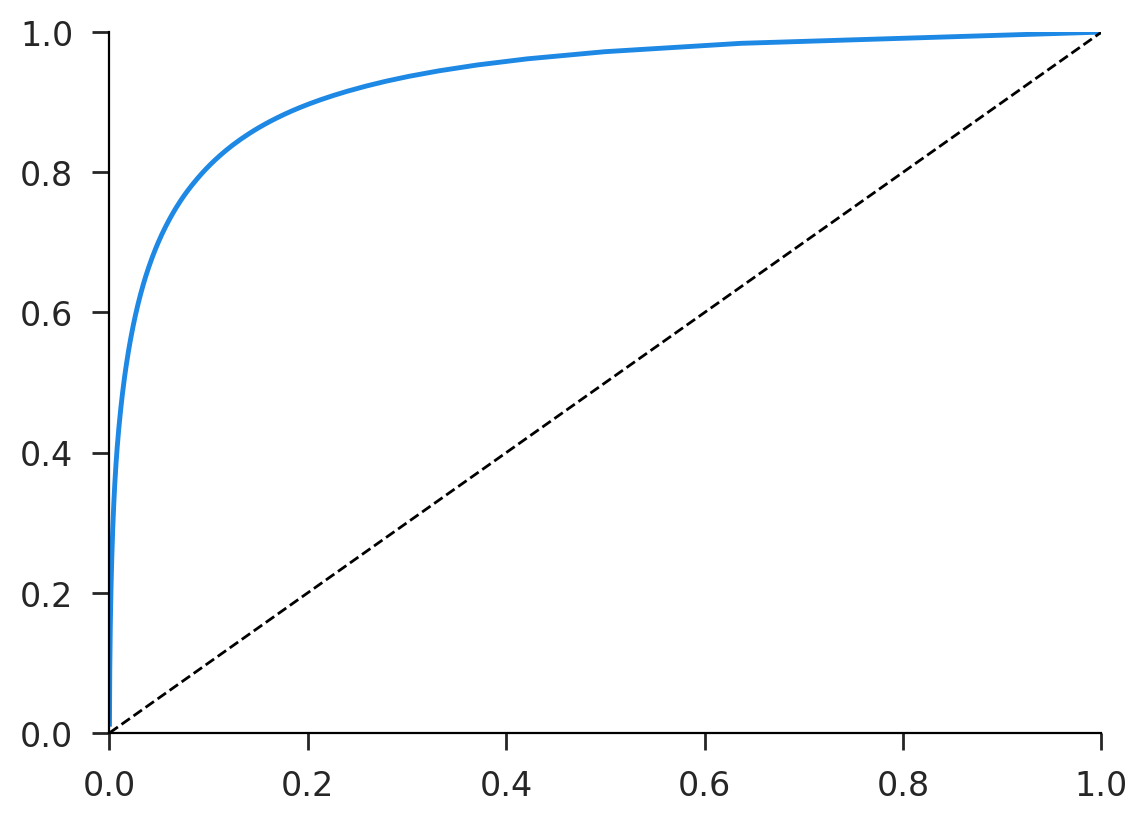In :
def word_gini(c):
n = len(c)
s = -np.sort(-c)
cdf = np.cumsum(s)/np.sum(c)
gini = 2*(np.sum(cdf)/n-0.5)
return gini

In :
ginis = [word_gini(counts[i,:].todense().getA1()) for i in range(nbook)]
info.assign(gini=ginis)

Out:
name length gini
0 austen-emma 887071 0.957791
1 austen-persuasion 466292 0.958783
2 austen-sense 673022 0.957912
3 bible-kjv 4332554 0.960545
4 blake-poems 38153 0.984016
5 bryant-stories 249439 0.970558
6 burgess-busterbrown 84663 0.989394
7 carroll-alice 144395 0.980115
8 chesterton-ball 457450 0.932614
9 chesterton-brown 406629 0.934194
10 chesterton-thursday 320525 0.946722
11 edgeworth-parents 935158 0.948896
12 melville-moby_dick 1242990 0.875626
14 shakespeare-caesar 112310 0.974411
15 shakespeare-hamlet 162881 0.958049
16 shakespeare-macbeth 100351 0.965897
17 whitman-leaves 711215 0.900018

### Text Visualization¶

In :
tsne = TSNE(init='random', perplexity=10)
embed = tsne.fit_transform(vecs)

In :
fig, ax = plt.subplots()
ax.scatter(*embed.T)
for i in range(nbook):
ax.annotate(
names[i], embed[i], xytext=(15, -7),
textcoords='offset pixels', fontsize=10
)